# NTSE Stage 1 - MAT (Mock Test 1)

## 50 Questions MCQ Test NTSE 2016 Mock Test Series for Class 10 (Stage 1) | NTSE Stage 1 - MAT (Mock Test 1)

Description
This mock test of NTSE Stage 1 - MAT (Mock Test 1) for Class 10 helps you for every Class 10 entrance exam. This contains 50 Multiple Choice Questions for Class 10 NTSE Stage 1 - MAT (Mock Test 1) (mcq) to study with solutions a complete question bank. The solved questions answers in this NTSE Stage 1 - MAT (Mock Test 1) quiz give you a good mix of easy questions and tough questions. Class 10 students definitely take this NTSE Stage 1 - MAT (Mock Test 1) exercise for a better result in the exam. You can find other NTSE Stage 1 - MAT (Mock Test 1) extra questions, long questions & short questions for Class 10 on EduRev as well by searching above.
QUESTION: 1

### Find the missing term in each of the following series 6, 11, 21, 36, 56, ?

Solution:

The pattern is + 5, + 10, + 15, + 20,....
.'. Missing Number = 56 + 25 = 81.

QUESTION: 2

### Find the missing term in each of the following series 4, 9, 25, ? , 121, 169, 289, 361

Solution:

The given series consists of squares of consecutive prime numbers
i.e. 22, 32, 52,.....,
112, 132, 172, 192.
So, missing term = 72 = 49.

QUESTION: 3

### Find the missing term in each of the following series 325, 259, 204, 160, 127, 105, ?

Solution:
QUESTION: 4

Find the missing term in each of the following series

5760, 960, ?, 48, 16, 8

Solution:
QUESTION: 5

Find the missing term in each of the following series

9, 27, 31, 155, 161, 1127, ?

Solution:
QUESTION: 6

Find the missing term in each of the following series

2, 3, 3, 5, 10, 13, ?, 43, 172, 177

Solution:
QUESTION: 7

Find the missing term in each of the following series

589654237, 89654237, 8965423, 965423, ?

Solution: The digits are removed one by one from the beginning and the end in order alternately. so as to obtain the subsequent terms of the series.
QUESTION: 8

Correct alternative that will same pattern and fill in the blank spaces.

90, 180, 12, 50, 100, 200, , 3, 50, 4, 25, 2, 6, 30, 3

Solution:

Clearly, 90 = 30 * 3,  180 = 6 * 30,  12 = 2 * 6,  50 = 25 * 2,  100 = 4 * 25,  200 = 50 * 4.
.'. Missing number = 3 * 50 = 150.

QUESTION: 9

Find the missing term in each of the following series

2, 12, 36, 80, 150, ?

Solution:
QUESTION: 10

In each of the following questions various terms of an alphabet series / letter number series given with one or more terms missing as shown by (?), choose the missing terms out of the given alternatives

Z, Y, X, U, T, S, P, O, N, K, ?, ?

Solution:
QUESTION: 11

In each of the following questions various terms of an alphabet series / letter number series given with one or more terms missing as shown by (?), choose the missing terms out of the given alternatives

ajs, gpy, ?, sbk, yhq

Solution:
QUESTION: 12

In each of the following questions various terms of an alphabet series / letter number series given with one or more terms missing as shown by (?), choose the missing terms out of the given alternatives

UPI, ?, ODP, MBQ, IAW

Solution:
QUESTION: 13

In each of the following questions various terms of an alphabet series / letter number series given with one or more terms missing as shown by (?), choose the missing terms out of the given alternatives

C4X, F9U, I16R, ?

Solution:
QUESTION: 14

In each of the following questions various terms of an alphabet series / letter number series given with one or more terms missing as shown by (?), choose the missing terms out of the given alternatives  J2Z, K4X, I7V, ?, H16R, M22P

Solution:
QUESTION: 15

In each of the following questions various terms of an alphabet series / letter number series given with one or more terms missing as shown by (?), choose the missing terms out of the given alternatives

Q1F, S2E, U6D, W21C, ?

Solution:
QUESTION: 16

In each of the following letter series sum of the letters are missing which are given in that order as one of the alternatives below it. Choose the correct alternatives.

ac __ cab __ baca __aba __ acac

Solution:
QUESTION: 17

In each of the following letter series sum of the letters are missing which are given in that order as one of the alternatives below it. Choose the correct alternatives.

__ bcc __ ac __ aabb __ ab __ cc

Solution:
QUESTION: 18

In each of the following letter series sum of the letters are missing which are given in that order as one of the alternatives below it. Choose the correct alternatives.

a __ bc __ c __ abb __ bca __

Solution:

The series is aa / b / cccc / a / bbbb / c / aa.

QUESTION: 19

In each of the following questions four words / numbers / group of letters have been given. Out of these three are alike in a certain way but the rest one is different. Choose the one which is different from the rest four.

Solution:
QUESTION: 20

In each of the following questions four words / numbers / group of letters have been given. Out of these three are alike in a certain way but the rest one is different. Choose the one which is different from the rest four.

Solution:
QUESTION: 21

In each of the following questions four words / numbers / group of letters have been given. Out of these three are alike in a certain way but the rest one is different. Choose the one which is different from the rest four.

Solution:
QUESTION: 22

In each of the following questions four words / numbers / group of letters have been given. Out of these three are alike in a certain way but the rest one is different. Choose the one which is different from the rest four.

Solution:
QUESTION: 23

In each of the following questions four words / numbers / group of letters have been given. Out of these three are alike in a certain way but the rest one is different. Choose the one which is different from the rest four.

Solution:
QUESTION: 24

In each of the following questions four words / numbers / group of letters have been given. Out of these three are alike in a certain way but the rest one is different. Choose the one which is different from the rest four.

Solution:
QUESTION: 25

In each of the following questions four words / numbers / group of letters have been given. Out of these three are alike in a certain way but the rest one is different. Choose the one which is different from the rest four.

Solution:
QUESTION: 26

In each of the following questions four words / numbers / group of letters have been given. Out of these three are alike in a certain way but the rest one is different. Choose the one which is different from the rest four.

Solution:
QUESTION: 27

In each of the following questions four words / numbers / group of letters have been given. Out of these three are alike in a certain way but the rest one is different. Choose the one which is different from the rest four.

Solution:
QUESTION: 28

In each of the following questions four words / numbers / group of letters have been given. Out of these three are alike in a certain way but the rest one is different. Choose the one which is different from the rest four.

Solution:
QUESTION: 29

The column and row of matrices I are numbered from 0 to 4 and those of matrix to from 5 to 9. A letter from these matrices can be represented first by its row and then the column number. Then identify the correct set for the word given in each question.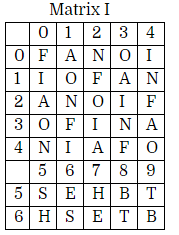Matrix II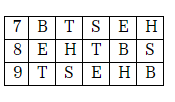NEST

Solution:
QUESTION: 30

The column and row of matrices I are numbered from 0 to 4 and those of matrix to from 5 to 9. A letter from these matrices can be represented first by its row and then the column number. Then identify the correct set for the word given in each question.Matrix IIFAITH

Solution:
QUESTION: 31

The column and row of matrices I are numbered from 0 to 4 and those of matrix to from 5 to 9. A letter from these matrices can be represented first by its row and then the column number. Then identify the correct set for the word given in each question.Matrix IIFINE

Solution:
QUESTION: 32

The column and row of matrices I are numbered from 0 to 4 and those of matrix to from 5 to 9. A letter from these matrices can be represented first by its row and then the column number. Then identify the correct set for the word given in each question.Matrix IIHEAT

Solution:
QUESTION: 33

If ‘rose’ is called ‘poppy’, ‘poppy’ is called ‘lily’, ‘lily’ is called ‘lotus’ and ‘lotus’ is called ‘gladiola’, which is the king of flowers?

Solution:

The king of flowers is the lotus But lotus is called gladiola.  So gladiola is the king of flowers.

QUESTION: 34

If ‘lead’ is called ‘stick’, ‘stick’ is called ‘nib’, ‘nib’ is called ‘needle’, ‘needle’ is called ‘rope’ and ‘rope’ is called ‘thread’, what will be fitted in a pen to write with it?

Solution:
QUESTION: 35

If air is called green , green is called blue, blue is called sky, sky is called yellow, yellow is called water and water is called pink, then what is the color of clear sky ?

Solution:

The colour of clear sky is 'blue' and as given, 'blue' is called 'sky'.
So, the colour of clear sky is 'sky'.

QUESTION: 36

If in a certain code, CERTAIN is coded as XVIGZRM, SEQUENCE is coded HVJFVMXV, then how would MUNDANE to coded?

Solution:
QUESTION: 37

Choose the number pair which is different from others

Solution:
QUESTION: 38

The town of Paranda is located on green lake. The town of Akram is west of Paranda. Tokhada is east of Akram but west of Paranda. Kakran is east of Bopri but west of Tokhada and Akram. If they are all in the same district, which town is the farthest west?

Solution:
QUESTION: 39

1        2      3

4        5      6

7        8      9

(27)   (38)  (?)

Solution:
QUESTION: 40

You are given the following situation. Select the most avoid action from the four alternatives. “when you get angry, you usually”

Solution:
QUESTION: 41

In each problem out of four figures marked (a), (b), (c), (d), three are similar in a certain manner. However one figures not like the other four. Choose the figure which is different from the rest.

Solution:
QUESTION: 42

Find the water image of a given figure (X)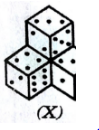Solution:
QUESTION: 43

Select from the alternatives, the box that can be formed by holding the sheet shown in figure (X)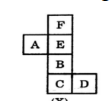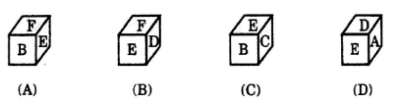Solution:
QUESTION: 44

In the given question from amongst the figures marked (1),(2),(3)and (4) , select the one which satisfies the same conductions of placement of the Dot as in figure (X).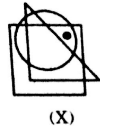Solution:
QUESTION: 45

In the matrix given below the values of A, B and C are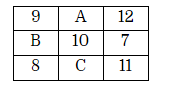Solution:
QUESTION: 46

Directions: each of the following questions consists of four problem figures marked A, B, C and D and four answer figures marked 1, 2, 3 and 4. Select a figure from amongst the answer figures which will continue the series established by the four problem figures.

PROBLEM FIGUER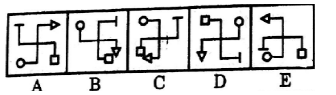Solution:
QUESTION: 47

Directions: each of the following questions consists of four problem figures marked A, B, C and D and four answer figures marked 1, 2, 3 and 4. Select a figure from amongst the answer figures which will continue the series established by the four problem figures.

PROBLEM FIGUER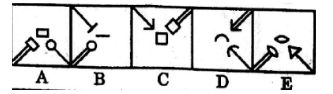Solution:
QUESTION: 48

Directions: each of the following questions consists of four problem figures marked A, B, C and D and four answer figures marked 1, 2, 3 and 4. Select a figure from amongst the answer figures which will continue the series established by the four problem figures.

PROBLEM FIGUER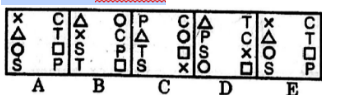Solution:
QUESTION: 49

Directions: in each problem out of four figures marked (a), (b), (c), (d), three are similar in a certain manner. However one figures not like the other four. Choose the figure which is different from the rest.

Solution:
QUESTION: 50

Directions: in each problem out of four figures marked (a), (b), (c), (d), three are similar in a certain manner. However one figures not like the other four. Choose the figure which is different from the rest.

Solution: